Next: 6.7 Calculation of Up: 6. Limits of Sequences Previous: 6.5 Illustrations of the   Index

# 6.6 Geometric Series

6.73   Theorem (Geometric series) Let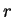be a real number such that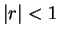. Then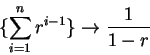(6.74)

Equation (6.74) is often written in the form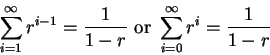Proof:    Letbe a real number such that, and for all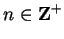let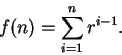Then by theorem 2.22 we have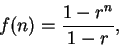and hence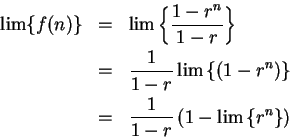Hence by the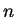th power theorem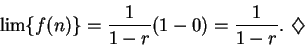6.75   Exercise. Find the error in the following argument. Let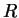be a real number with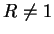, and forin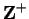, let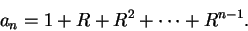Let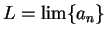. Then, by the translation rule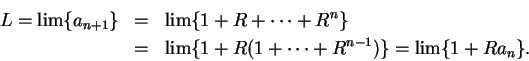Thus by the sum rule and product rule,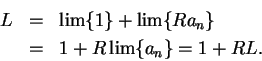Now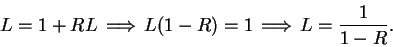Hence we have shown that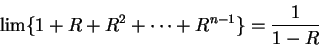for all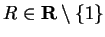. (This sort of argument, and the conclusion were regarded as correct in the eighteenth century. At that time the argument perhaps was correct, because the definitions in use were not the same as ours.)

The clearest early account of the summation of geometric series''[6, page 136] was given by Grégoire de Saint-Vincent in 1647. Grégoire's argument is roughly as follows: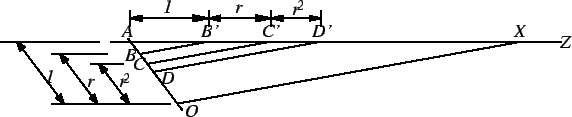On the line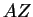mark off points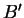,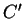,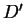etc. such that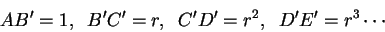On a different line through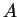mark off points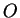,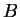,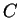,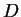etc. such that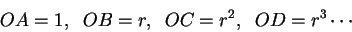Then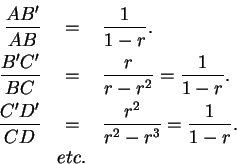Now I use the fact that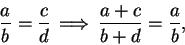(6.76)

(see exercise 6.78), to say that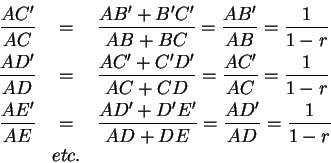It follows that the triangles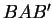,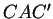,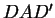, etc. are all mutually similar, so the lines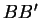,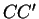,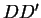etc. are all parallel. Draw a line throughparallel toand intersectingat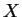. I claim that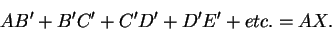(6.77)

It is clear that any finite sum is smaller that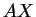, and by taking enough terms in the sequence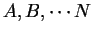we can make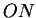arbitrarily small. Then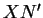is arbitrarily small, i.e. the finite sums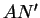can be made as close toas we please. By similar triangles,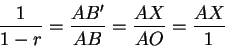so, equation (6.77) says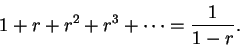6.78   Exercise. Prove the assertion (6.76).

6.79   Exercise.
a)
Find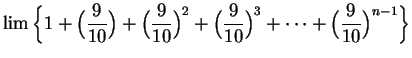.
b)
Find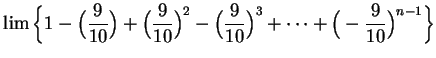.
c)
For eachinlet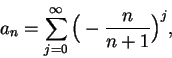(in part (b) you calculated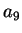). Find a formula for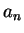, and then find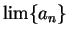.
d)
Show that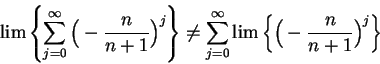(6.80)

(Thus it is not necessarily true that the limit of an infinite sum is the infinite sum of the limits. The left side of (6.80) was calculated in part c. The right side is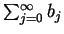, where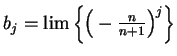depends on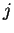, but not on.)Next: 6.7 Calculation of Up: 6. Limits of Sequences Previous: 6.5 Illustrations of the   Index
Ray Mayer 2007-09-07